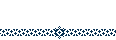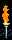# Code generator for GameBoy version of Prince of PersiaA code for Prince of Persia on the GameBoy consists of eight numbers. In these numbers is stored the number of lives you have, the level you're in and the amount of time (only in minutes) you have left. This information is stored in the eight numbers as follows (if you just want the codes you can use the form below:
Digit 1: (Level mod 10 + Level div 10 + Lives) mod 10 (Time mod 10 + Time div 10) mod 10 (Lives mod 10 + Level div 10) mod 10 Lives Time mod 10 Time div 10 Level mod 10 Level div 10

The meaning of the words mod and div are as follows:
mod 10 means what's left after you've taken all multiples of ten, for example 47 mod 10 = 7 and 68 mod 10 = 8,
div 10 means the number of multiples of ten that are in a number, for example 47 div 10 = 4 and 68 div 10 = 6.

After calculating these numbers, you should encode them according to the following translation table:
 0 1 2 3 4 5 6 7 8 9 5 4 7 6 1 0 3 2 9 8

Here's a piece of JavaScript that does the work for you:
 Level: Number of lives: Time left: Code:

You can also use this the other way around, to see from a code you've got on a paper somewhere what time you had left after each level. You can use the above method for this, or you could use the form below:
 Code: Level: Number of lives: Time left:

We would like to thank Ewoud Dronkert and Roy de Boer for their help figuring out how these codes work.### Jan and Rob.

Back to the main page